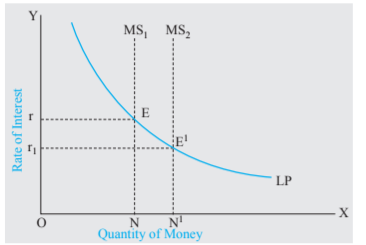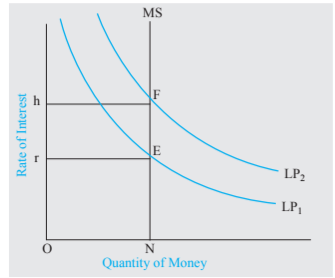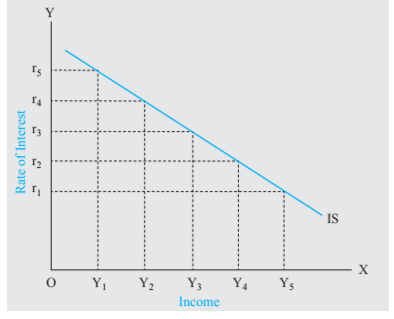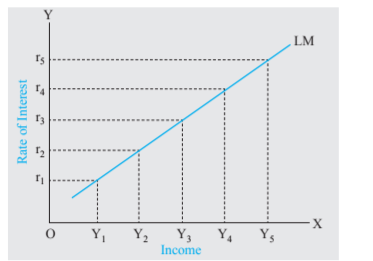# JAIIB IE and IFS Paper-1 Module-B Unit 4: Theory of Interest

## JAIIB Paper 1 (IE and IFS) Module B Unit 4: Theory of Interest (New Syllabus)

IIBF has released the New Syllabus Exam Pattern for JAIIB Exam 2023. Following the format of the current exam, JAIIB 2023 will have now four papers. The JAIIB Paper 1 (Indian Economy & Indian Financial System) includes an important topic called “Theory of Interest”. Every candidate who are appearing for the JAIIB Certification Examination 2023 must understand each unit included in the syllabus. In this article, we are going to cover all the necessary details of JAIIB Paper 1 (IE and IFS) Module B ECONOMIC CONCEPTS RELATED TO BANKING Unit 4: Theory of Interest Aspirants must go through this article to better understand the topic, Theory of Interest and practice using our Online Mock Test Series to strengthen their knowledge of Banker Customer Relationship. Unit 4: Theory of Interest

### Interest

Interest is a payment made by a borrower for the use of a sum of money for a period of time; it is one of the four types of income, the others being rent, wages, and profit. Three elements can be distinguished in interest:

• Payment for the risk involved in making the loan;
• Payment for the trouble involved;
• Pure interest, that is a payment for the use of the money.

### Theory of Interest

It is also known as the demand and supply theory. It was developed and modified by various renowned economists like Marshall, Fisher, and Pigou. According to this theory, rate of interest is determined by the equilibrium of demand and supply of savings. The classical economists maintained that interest is a price paid for the supply of savings, to meet the demand for investment.

#### Demand for Savings

• Demand for savings comes from investors for investment in business activities. The demand for investment is high when the capital, as a factor of production, give high returns.
• The demand for capital, or investment demand, is determined by the productivity of capital, or returns on investment, on the one hand, and the rate of interest, or the cost of investment, on the other.

#### Supply of Savings

• The supply of capital is determined by savings, which are determined by a range of psychological, economic, and institutional elements. Savings depend on various factors like capacity to save, willingness to save, income level, etc.
• Saving entails reducing consumption or deferring current spending. Saving entails, a sacrifice, abstinence, or patience. As savings is an incentive to follow the act of savings or refrain from current spending, when selecting between present consumption (which requires no saving) and future consumption (which requires saving), the person must consider the opportunity cost of each  choice, which is quantified by the rate of interest.

#### Equilibrium of Demand and Supply of savings

• For a particular level of income, the supply of savings increases with increase in interest rate but this increase in interest rates decreases the demand for saving by the borrowers.
• This decrease in demand results in interest rates going down, which in turn, reduces the supply.

#### Criticism to the Classical

• Theory of Interest Keynes questioned the classical theory of interest on several grounds. Interest, according to Keynes, is not a reward for saving, rather, it is a monetary phenomenon, and the rate of interest is defined as a reward for parting with liquidity (or cash balances), rather than a reward for saving.
• Keynes objected to the classical theory that saving and investment are interest elastic. He stated that, in actuality, investment is more dependent on the marginal efficiency of capital and future expectations than on interest rates, particularly during times of economic slowdown.

### Keynes’ Liquidity Preference Theory Of Rate Of Interest

Liquidity Preference Theory suggests that investors demand progressively higher premiums on medium and long-term securities as opposed to short-term securities. According to the theory, which was developed by John Maynard Keynes in support of his idea that the demand for liquidity holds speculative power, liquid investments are easier to cash in for full value.

Cash is commonly accepted as the most liquid asset. According to the liquidity preference theory, interest rates on short-term securities are lower because investors are not sacrificing liquidity for greater time frames than medium or longer-term securities.

#### Special Considerations

• Keynes introduced Liquidity Preference Theory in his book The General Theory of Employment, Interest and Money. Keynes describes the theory in terms of three motives that determine the demand for liquidity:
• The transactions motive states that individuals have a preference for liquidity to guarantee having sufficient cash on hand for basic day-to-day needs. In other words, stakeholders have a high demand for liquidity to cover their short-term obligations, such as buying groceries and paying the rent or mortgage. Higher costs of living mean a higher demand for cash/liquidity to meet those day-to-day needs.

#### Precautionary motive

• The precautionary motive relates to an individual’s preference for additional liquidity if an unexpected problem or cost arises that requires a substantial outlay of cash. These events include unforeseen costs like house or car repairs.

#### Speculative Motive

• Stakeholders may also have a speculative motive. When interest rates are low, demand for cash is high and they may prefer to hold assets until interest rates rise. The speculative motive refers to an investor’s reluctance to tying up investment capital for fear of missing out on a better opportunity in the future.

### Money Demand Curve

• It follows from above that quantity of money demanded increases with the fall in the rate of interest or with the increase in level of nominal income. At a given level of nominal income, we can draw a money demand curve showing the quantity of money demanded at various rates of interest.
• As demand for money is inversely related to the rate of interest, the money demand curve at a given level of income, will be downward-sloping, as is shown by the curve LP1 in Figure. When the level of money income increases, the curve of demand for money shifts upward to the new position LP2.### Effect Of An Increase In The Money Supply

Let us now examine the effect of increase in money supply on the rate of interest. In Figure, LP is the money demand curve for satisfying various motives. To begin with, ON is the quantity of money available. Rate of interest will be determined where the demand for money is in balance or equal to the fixed supply of money ON. It is clear from the figure that demand for money is equal to ON quantity of money at ‘Or’ rate of interest. Hence, ‘Or’ is the equilibrium rate of interest. Assuming no change in  expectations and nominal income, an increase in the quantity of money (through buying securities by  the central bank of the country from the open market), will lower the rate of interest. In Figure, when the quantity of money increases from ON to ON1, the rate of interest falls from Or to Or1, because the  new quantity of money ON1 is in balance with the demand for money at Or1 rate of interest. In this case, we move down on the curve. Thus, given the money demand curve or curve of liquidity preference, an increase in the quantity of money brings down the rate of interest.  Let us see how increase in money supply leads to the fall in the rate of interest. With initial equilibrium at Or, when the money supply is expanded from ON to ON1, there emerges excess supply of money at the  initial ‘Or’ rate of interest. The people would react to this excess quantity of money supplied by buying bonds. As a result, the bond prices will go up which implies that the rate of interest declines. This is how the increase in money supply leads to the fall in rate of interest.### Shifts In Money Demand Or Liquidity Preference Curve

The position of money demand curve depends upon two factors: (1) the level of nominal income and (2) the expectations about the changes in bond prices in the future, which implies change in rate of interest  in future. As has been explained above, a money demand curve is drawn by assuming a certain level of nominal income. With the increase in nominal income, money demand for transactions and precautionary  motives increases, causing an upward shift in the money demand curve.Shifts in money demand curve (or what Keynes called liquidity preference curve) can also be caused  by changes in the expectations of the people, regarding changes in bond prices or movements in the rate  of interest, in future. If some changes in events lead the people on balance to expect a higher rate of interest in the future than they had previously supposed, the money demand or liquidity preference for speculative motive will increase, which will bring about an upward shift in the money demand curve or  liquidity preference curve and this will raise the rate of interest.  In Figure, assuming that the quantity of money remains unchanged at ON, the rise in the money demand or liquidity preference curve from LP1 to LP2, the rate of interest rises from Or to Oh because at  Oh, the new speculative demand for money is in equilibrium with the supply of money ON. It is worth noting that when the liquidity preference curve rises from LP1 to LP2, the amount of money held does not increase; it remains ON as before. Only the rate of interest rises from Or to Oh to equilibrate the new liquidity preference or money demand with the available quantity of money ON.

### Hansen Synthesis: IS-LM Curve Model

Renowned economists, Sir John Richard Hicks and Alvin Hansen, have brought about a synthesis between the Classical and Keynes’ theories of interest and have thereby succeeded in propounding an adequate  and determinate theory of interest. This involves three steps viz.

I) Using Classical Theory, to derive a curve called IS Curve. (IS- Investment Savings)

II)Using Keynes’ Theory, to derive a curve called LM curve (LM-Liquidity-Preference-Money Supply)

III)Combining IS and LM curves.

#### Using Classical Theory to Derive IS Curve

From the classical theory we get a family of saving curves at various income levels, as shown in Fig 1. These are represented by S1Y1, S2Y2, S3Y3, S4Y4 and S5Y5. As the income rises, the savings curve shifts to the right, which means that as the income rises, the savings also rise, provided the interest  remains constant. The other curve shown in Fig. I is the investment curve and is marked as I. It shows that the investment demand increases as the interest rate falls. This investment curve intersects the savings curves and the points of intersection represent the rates of interest, which equalise savings and  investment at various income levels. Thus, when income is Y2, the relevant savings curve is S2Y2 and the corresponding rate that equalises savings and investment is r4. Similarly, for other levels of income rates of interest that equalise savings and investment can be obtained. We observe that the interest rate which equalises savings and investments, keeps on falling as the income level increases. In Fig.2, we plot this co-relation between income (on the X-axis) and the corresponding rates of interest determined by  the equality of savings and investment (on the Y-axis), by taking these points of intersection from Fig.1. The resulting curve is marked as IS curve. Since, as income increases, rate of interest falls, the IS curve slopes downward. Fig.1 and Fig.2 are both based on the Classical Theory explained earlier.  The steepness of the IS curve depends upon the elasticity or sensitiveness of investment demand to the changes in rate of interest. When investment demand is greatly elastic or highly sensitive to the rate of interest, the IS curve will be flat (i.e., less steep). On the other hand, when investment demand is not very sensitive to the changes in rate of interest, the IS curve will be relatively steep.Figure 1: Derivation of IS Curve – Interest Rate vs Saving and InvestmentFigure 2: Derivation of IS Curve – Interest Rate vs Income

#### Using Keynes’ Theory to Derive LM Curve

The LM curve is derived from the Keynesian liquidity preference theory of interest. This is depicted in  Fig. (a) and (b). Fig. (a) shows various liquidity preference curves at various levels of income.  The greater the level of income, the greater is the amount of money held for transactions motive and,  herefore, the higher the level of liquidity preference curve. Now, the intersection of these various liquidity preference curves, with the supply curve of money supply fixed by the monetary authority (represented by  line NS), would give us the rates of interest which equalise the demand for money (liquidity preference)  to the supply of money at various levels. We observe from Fig.(a) that the interest rate at which the equilibrium of liquidity preference and money supply is achieved, keeps on increasing as the income  level goes up. All these points of intersection in Fig. (a) are used to plot curve shown as LM in Fig.  (b). Each point on the LM curve points to a rate of interest at which, the equilibrium of liquidity preference and money supply is achieved, at a particular level of income.  Figure (b) shows that the LM curve slopes upward to the right. This is because with higher levels of income, demand for money (that is, the liquidity preference) is higher and consequently, the moneymarket equilibrium, that is, the equality of the given money supply with liquidity preference curve occurs,  at a higher rate of interest. This implies that rate of interest varies directly with income. It is important to know which factors determine the slope of the LM curve. There are two factors on which the slope of the LM curve depends. First, the responsiveness of demand for money (i.e., liquidity preference) to the changes in income. As the income increases, say from Yl to Y2, the liquidity preference curve shifts from LPl to LP2, that is, with an increase in income, demand for money would increase for being held for  transactions motive, L = f(Y). This extra demand for money would disturb the money-market equilibrium, and in order to restore the equilibrium, the rate of interest will rise to the level where the given money  supply curve intersects the new liquidity preference curve corresponding to the higher income level.Figure (a) Determination of LM Curve from Keynes’ Liquidity Preference Curves – Interest Rate vs Income.Figure (b): Determination of LM Curve from Keynes’ Liquidity Preference Curves – Interest Rate vs Income

#### Combining IS and LM Curves

The IS curve and the LM curve relate the two variables: (a) income, and (b) the rate of interest. These curves are plotted in Fig.2 We note that the IS curve, which represents Classical Theory, slopes downwards while the LM curve, which represents the Keynes’ Theory, moves upwards. The intersection of these two curves represents the synthesis of the two theories. The point of intersection of these two curves, i.e., E in Fig. 2, represents the equilibrium rate of interest.Figure 2: Intersection of the IS and LM Curves

The equilibrium rate of interest thus determined is Or3 and the level of income determined is OY3. At  this point, income and the rate interest stand in relation to each other such that (1) investment and saving  are in equilibrium, and (2) the demand for money is in equilibrium with the supply of money (i.e., the  desired amount of money is equal to the actual supply of money). It should be noted that LM curve has been drawn by taking the supply of money as fixed.  Thus, a determinate theory of interest is based on: (1) the investment-demand function, (2) the saving function (or, conversely, the consumption function), (3) the liquidity preference function, and (4) the  quantity of money supply. We see, therefore, that according to Hicks and Hansen, both monetary and real factors, namely, productivity, thrift, and the monetary factors, that is, the demand for money (liquidity  preference) and supply of money play a part in determining of the rate of interest. Any change in these factors will cause shift in IS or LM curve and will, therefore, change the equilibrium level of the rate of  interest and income.

JAIIB IE & IFS Paper-1 Module-B Unit-4 Theory of Interest PDF